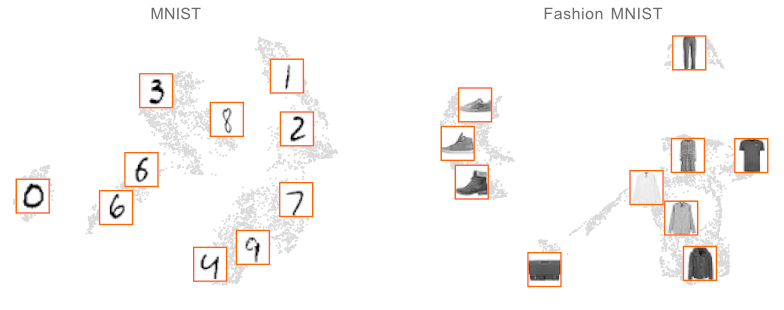# Data summarization

## On maps drawn at smaller than 1:1 scaleSummary statistics which don’t require you to keep all the data but which allow you to do inference nearly as well. e.g sufficient statistics in exponential families allow you to do certain kind of inference perfectly without anything except summaries. Methods such as variational Bayes summarize data by maintaining a posterior density (usually a mixture models) as a summary of all the data, at some cost in accuracy. I think of these as nearly sufficient statistics but there are other framings, as data summarization which I am going to note here for later reference.

• Approximate Bayesian Computation
• inducing sets, as seen in Gaussian processes
• coresets as seen in Bayesian linear models
• probabilistic deep learning possibly does this
• Bounded Memory Learning considers this from a computation complexity standpoint - which hypotheses can be learned from data subsets

TBC.

## Coresets

Bayesian. Solve an optimisation problem to minimise distance between posterior with all data and with a weighted subset.

https://github.com/trevorcampbell/bayesian-coresets

## representative subsets

I think this is intended to be generic? See apricot:apricot implements submodular optimization for the purpose of summarizing massive data sets into minimally redundant subsets that are still representative of the original data. These subsets are useful for both visualizing the modalities in the data (such as in the two data sets below) and for training accurate machine learning models with just a fraction of the examples and compute.

## Directly approximate log likelihood

### No comments yet. Why not leave one?

GitHub-flavored Markdown & a sane subset of HTML is supported.